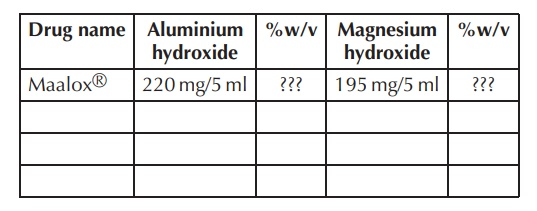# Exercises - The Boron Group - Group 13

| Home | | Inorganic Pharmaceutical Chemistry |

## Chapter: Essentials of Inorganic Chemistry : The Boron Group - Group 13

Inorganic Chemistry : The Boron Group - Group 13: Exercises

Exercises

1. Draw the Lewis structure or chemical formula of the following aluminium-based drugs

(a)        Aluminium acetate

(b)       Aluminium chloride

(c)        Aluminium oxide

2. Research different antacids mixtures, state their content and calculate the weight/volume per-centage (%w/v) for each active pharmaceutical ingredient (API).3. A typical antacid capsule contains 475 mg aluminium hydroxide as the active ingredient.

How many milligrams of stomach acid (HCl) can be neutralised by one tablet?

4. An aluminium hydroxide suspension (30 ml) containing 500 mg/5 ml aluminium hydroxide is prescribed to the patient. The prescription states the patient has to take 30 ml four times a day.

(a)        What is the chemical formula of aluminium hydroxide?

(b)       How many grams of Al3+ is given to the patient per single dose?

(c)        What is the weight/volume percentage (%w/v) of aluminium hydroxide in the suspension?

5. Write the chemical equations explaining the amphoteric behaviour of gallium hydroxide.